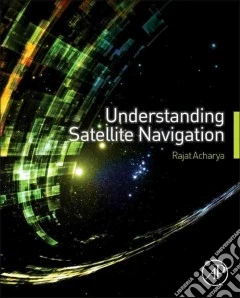ricerca
avanzata

## Un libro in lingua di Rajat Acharya edito da Academic Pr, 2014

• € 107,70
• Il prezzo è variabile in funzione del cambio della valuta d’origineThis book explains the basic principles of satellite navigation technology with the bare minimum of mathematics and without complex equations. It helps you to conceptualize the underlying theory from first principles, building up your knowledge gradually using practical demonstrations and worked examples. A full range of MATLAB simulations is used to visualize concepts and solve problems through simulations, allowing you to see what happens to signals and systems with different configurations. Implementation and applications are discussed, along with some special topics such as Kalman Filter and Ionosphere.

With this book you will learn:

• How a satellite navigation system works
• How to improve your efficiency when working with a satellite navigation system
• How to use MATLAB for simulation, helping to visualize concepts
• Various possible implementation approaches for the technologyThe most significant applications of satellite navigation systems
• Teaches the fundamentals of satellite navigation systems, using MATLAB as a visualization and problem solving tool
• Worked out numerical problems are provided to aid practical understanding
• On-line support provides MATLAB scripts for simulation exercises and MATLAB based solutions, standard algorithms, and PowerPoint slides